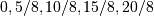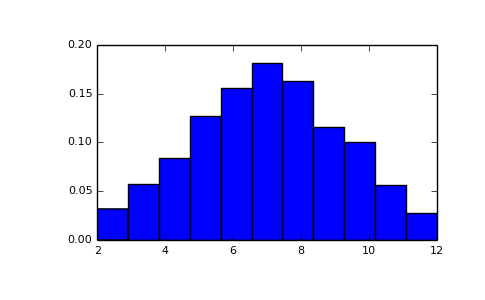# numpy.random.random_integers¶

numpy.random.random_integers(low, high=None, size=None)

Return random integers between low and high, inclusive.

Return random integers from the “discrete uniform” distribution in the closed interval [low, high]. If high is None (the default), then results are from [1, low].

Parameters: low : int Lowest (signed) integer to be drawn from the distribution (unless high=None, in which case this parameter is the highest such integer). high : int, optional If provided, the largest (signed) integer to be drawn from the distribution (see above for behavior if high=None). size : int or tuple of ints, optional Output shape. If the given shape is, e.g., (m, n, k), then m * n * k samples are drawn. Default is None, in which case a single value is returned. out : int or ndarray of ints size-shaped array of random integers from the appropriate distribution, or a single such random int if size not provided.

random.randint
Similar to random_integers, only for the half-open interval [low, high), and 0 is the lowest value if high is omitted.

Notes

To sample from N evenly spaced floating-point numbers between a and b, use:

```a + (b - a) * (np.random.random_integers(N) - 1) / (N - 1.)
```

Examples

```>>> np.random.random_integers(5)
4
>>> type(np.random.random_integers(5))
<type 'int'>
>>> np.random.random_integers(5, size=(3.,2.))
array([[5, 4],
[3, 3],
[4, 5]])
```

Choose five random numbers from the set of five evenly-spaced numbers between 0 and 2.5, inclusive (i.e., from the set):

```>>> 2.5 * (np.random.random_integers(5, size=(5,)) - 1) / 4.
array([ 0.625,  1.25 ,  0.625,  0.625,  2.5  ])
```

Roll two six sided dice 1000 times and sum the results:

```>>> d1 = np.random.random_integers(1, 6, 1000)
>>> d2 = np.random.random_integers(1, 6, 1000)
>>> dsums = d1 + d2
```

Display results as a histogram:

```>>> import matplotlib.pyplot as plt
>>> count, bins, ignored = plt.hist(dsums, 11, normed=True)
>>> plt.show()
```#### Previous topic

numpy.random.randint

#### Next topic

numpy.random.random_sample